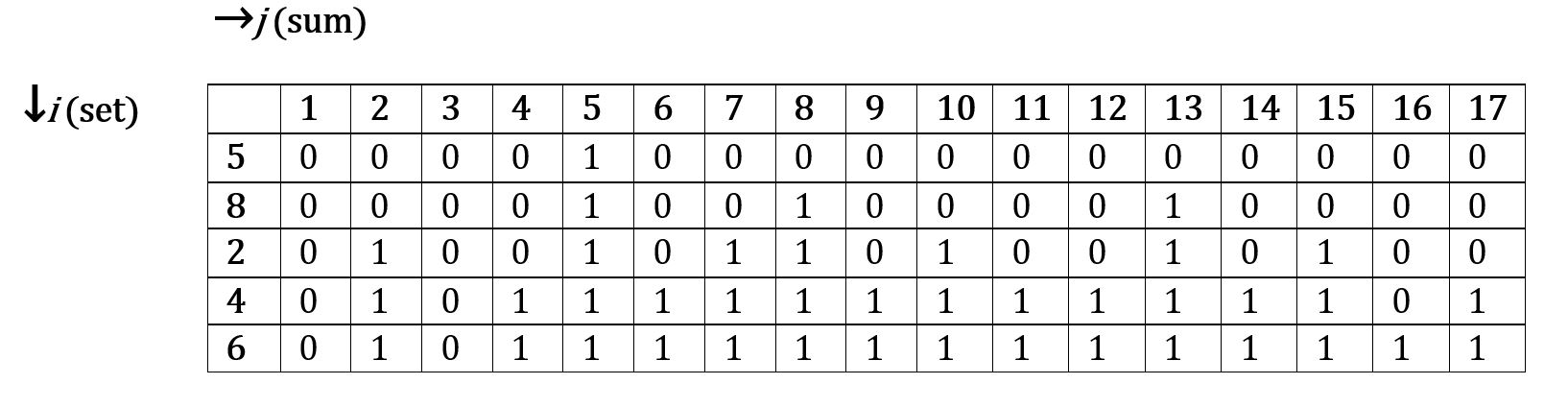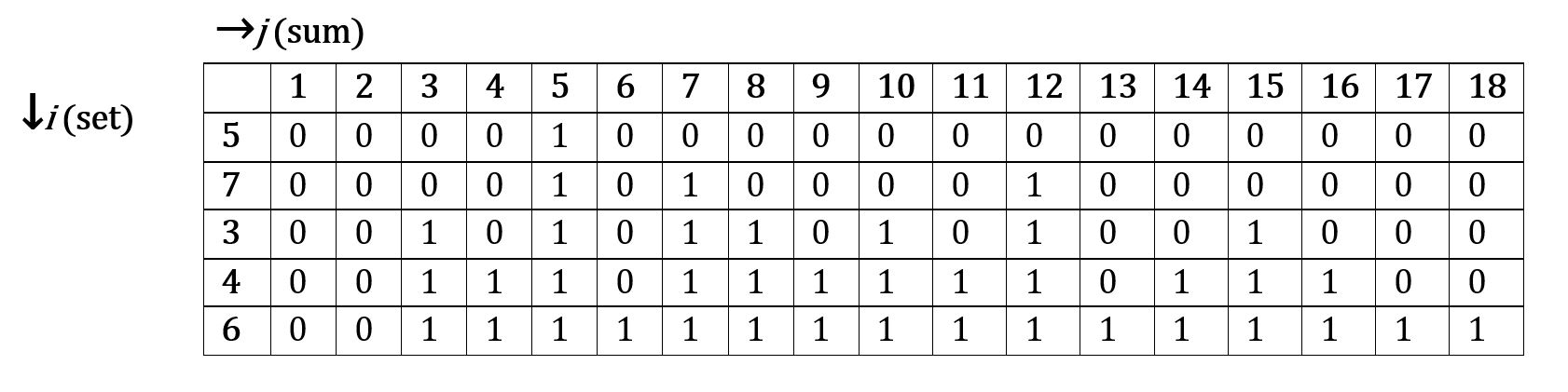CoCalc Public Filesprojects_F20 / dyn_Proj10 / sum finder.ipynb
Author: Julia Burnside
Views : 136
Compute Environment: Ubuntu 20.04 (Default)

# The Sum Finder Problem

A $2D$ array $m[ i ][ j ]$ is created with $i=(length\ of\ set)$ multiplied by $j=(size\ of\ the\ target\ value)$.

If there exists a subset of elements in $m[ i ]$ from $i = 0\ to\ i$, whose sum is equal to the value of $m[ j ]$;

$m[ i ][ j ] =1$.

Else, $m[ i ][ j ] = 0$.

If the value in the $m[ i-1 ][ j ]$ row is $1$, we can immediately evaluate the $m[0\ to\ i ][ j ]$ row to $1$ without any extraneous calculations, since we already know a subset of values equal to the value of $m[ i ][ j ]$ exists.

###### Set = $[5, 8, 2, 4, 6]$For the first row $i$, we only have one value in our subset; $$.

So only the array element holding the value of $j=$ will be appended to $1$. The rest are $0$.

For the second row, $i$, we have two values in our subset; $[5, 8]$.

So the array element holding the values of $j=[8, 5+8=13]$ will be appended to $1$. The rest are $0$.

Since we have already evaluated the elements in the $m[i-1][j]$ position, we no longer need to evaluate the previous row and column numbers for values which have already been appended to $1$ but rather can continue to place a $1$ in all the remaining $m[0\ to\ i][j]$ positions. We only need to evaluate the new additions to our subset.

For the third row, $i$, we have three values in our subset; $[5, 8, 2]$.

$j=[5, 8, 13]$ have already been evaluated. So, we only need to evaluate the addition of $2$ to the subset. The array elements holding the values of $j=[2, 5+2=7, 8+2=10, 5+8+2=15]$ need to be appended to $1$. The rest are $0$.

For the fourth row, $i$, we have four values in our subset; $[5, 8, 2, 4]$.

$j=[2, 5, 7, 8, 10, 13, 15]$ have already been evaluate. So, we only need to evaluate the addition of $4$ to the subset. The array elements holding the values of $j=[4, 4+2=6, 4+5=9, 4+2+5=11, 4+8=12, 4+2+8=14 4+8+5=17]$ need to be appended to $1$. The rest are $0$.

Furthermore, we have already evaluated the element holding the value of $j=[2, 5, 7, 8, 10, 13]$ to be $1$, so the additional sum of $4$ to any of these values in our subset will append the values in the positions of the values $j=[2+4, 5+4, 7+4, 8+4, 10+4, 13+4]$ to all be $1$.

We already have our target value of $17$. But for the sake of this exercise, lets evaluate the final row.

For the fifth row, $i$, we have all five values in our subset; $[5, 8, 2, 4, 6.]$.

All other values in $j$ have been appended to $1$ with the exception of the values of $j=[1, 3, 16]$. We have already evaluated the value of $j=$ to be $1$, so the addition of 6 will guarantee that some combination of the subset will equal a sum of $16$, so we appended it to $1$. The others remain $0$.

###### Set = $[5, 7, 3, 4, 6]$For the first row $i$, we only have one value in our subset; $$.

So only the array element holding the value of $j={5}$ will be appended to $1$. The rest are $0$.

For the second row, $i$, we have two values in our subset; $[5, 7]$.

So the array element holding the values of $j=[7, 5+7=12]$ will be appended to $1$. The rest are $0$.

For the third row, $i$, we have three values in our subset; $[5, 7, 3]$.

$j=[5, 7, 12]$ have already been evaluated. So, we only need to evaluate the addition of $3$ to the subset. The array elements holding the values of $j=[3, 5+3=8, 7+3=10, 5+7+3=15]$ need to be appended to $1$. The rest are $0$.

For the fourth row, $i$, we have four values in our subset; $[5, 7, 3, 4]$.

$j=[3, 5, 7, 8, 10, 12, 15]$ have already been evaluate. So, we only need to evaluate the addition of $4$ to the subset. The array elements holding the values of $j=[4, 4+5=9, 7+4=11, 7+3+4= 14, 3+4+5+7=16]$ need to be appended to $1$. The rest are $0$.

Furthermore, we have already evaluated the element holding the value of $j=[5, 7, 10 , 12]$ to be $1$, so the additional sum of $4$ to any of these values in our subset will append the values in the positions of the values $j=[5+4, 7+4, 10+4, 12+4]$ to all be $1$.

For the fifth row, $i$, we have all five values in our subset; $[5, 7, 3, 4, 6]$.

All other values in $j$ have been appended to $1$ with the exception of the values of $j=[1, 2, 6, 13, 17, 18]$. We have already evaluated the value of $j=[7, 11, 12]$ to be $1$, so the addition of $6$ will guarantee that some combination of the subset will equal a sum of $j=[6, 13, 17, 18]$ so we appended them to $1$. The others remain $0$.